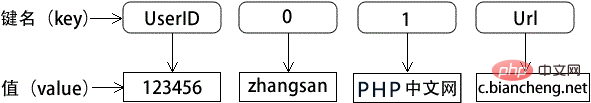# php数组有哪几种类型

php数组的类型：1、索引数组，其下标（键名）由数字组成，默认从0开始，每个数字对应一个数组元素在数组中的位置；2、关联数组，其下标（键名）由数值和字符串混合的形式组成；3、多维数组，包含一个或多个数组的数组。PHP 数组比其他高级语言中的数组更加灵活，不但支持以数字为键名的索引数组，而且支持以字符串或字符串、数字混合为键名的关联数组。而在其他高级语言中，如 Java 或者 C++ 等语言的数组，只支持数字索引数组。

PHP 数组的结构如下图所示：php数组的类型

• 索引数组：数组的下标是数字；

• 关联数组：数组的下标为字符是关联数组；

• 多维数组：包含一个或多个数组的数组；

1、索引数组

```<?php
//创建一个索引数组，索引数组的键是“0”，值是“苹果”
\$fruit=array("苹果","香蕉");
print_r(\$fruit);
?>```

```Array
(
 => 苹果
 => 香蕉
)```

• `array='苹果';`

• `\$arr=array('0'=>'苹果');`

• `\$arr=array('苹果');`

```<?php
//请创建一个数组变量arr,并尝试创建一个索引数组，键是0，值是苹果
\$arr=array(0=>'苹果');
if( isset(\$arr) ) {print_r(\$arr);}
?>```

```<?php
\$fruit=array('苹果','香蕉','菠萝');
foreach(\$fruit as \$key=>\$value){
echo '<br>第'.\$key.'值是：'.\$value;
}

?>```

```第0值是：苹果

2、关联数组

“关联数组”是一种具有特殊索引方式的数组。使用字符串或者其他类型的值（除了NULL）来索引它。

```<?php
//创建一个关联数组，关联数组的键“orange”，值是“橘子”
\$fruit=array('orange'=>'橘子');
echo \$fruit['orange'];
?>```

3、多维数组

```<?php
\$arr = array(
'安徽' => array(
'阜阳'=>array('阜南县','临泉县','颍州区'),
'宿州'=>array('墉桥区','灵璧县','泗县'),
'合肥'=>array('蜀山区','长丰县','肥东')
),
'河南' => array(
'洛阳'=>array('西工区','老城区','孟津县'),
'郑州市'=>array('中原区','金水区')
)
);
print_r(\$arr);
echo \$arr['安徽']['宿州'];  // 输出墉桥区
?>```

```Array
(
[安徽] => Array
(
[阜阳] => Array
(
 => 阜南县
 => 临泉县
 => 颍州区
)
[宿州] => Array
(
 => 墉桥区
 => 灵璧县
 => 泗县
)
[合肥] => Array
(
 => 蜀山区
 => 长丰县
 => 肥东
)
)
[河南] => Array
(
[洛阳] => Array
(
 => 西工区
 => 老城区
 => 孟津县
)
[郑州市] => Array
(
 => 中原区
 => 金水区
)
)
)### 全部评论我要评论

• 取消发布评论发送
• 1/1